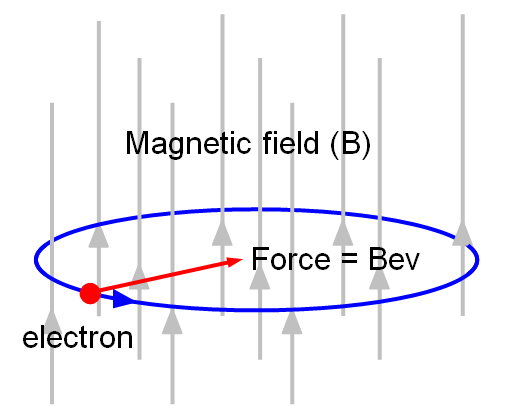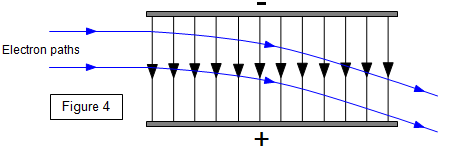# Motion in magnetic and electric fields

## Magnetic fields

The force (F) on wire of length L carrying a current I in a magnetic field of strength B is given by the equation:

F = BIL

But Q = It and since Q = e for an electron and v = L/t you can show that :
Magnetic force on an electron = BIL = B[e/t][vt] = Bev where v is the electron velocity

In a magnetic field the force is always at right angles to the motion of the electron (Fleming's left hand rule) and so the resulting path of the electron is circular (Figure 1).Therefore :

Magnetic force = Bev = mv2/r = centripetal force

v = [Ber]/m

and so you can see from these equations that as the electron slows down the radius of its orbit decreases.

Charged particles move in circles at a constant speed if projected into a magnetic field at right angles to the field.
Charged particles move in straight lines at a constant speed if projected into a magnetic field along the direction of the field.Figure 2 shows a 3D diagram of and electron moving at right angles to a uniform magnetic field.

If the electron enters the field at an angle to the field direction the resulting path of the electron (or indeed any charged particle) will be helical as shown in figure 3. Such motion occurs above the poles of the Earth where charges particles from the Sun spiral through the Earth's field to produce the aurorae.## Path of the electron in an electric field

We will consider next the case of an electron entering a uniform electroc field between two parallel plates (Figure 4). The potential difference between the plates is V and the plates are aligned along the x direction and the electron enters the field at right angles to the field lines:
The force on the electron is given by the equation:

F = eE = eV/d = maBut since there is a force the electron must accelerate in the y direction and the acceleration is given by a = 2y/t2. (From the equation s = y = ut + ˝ at2)

Therefore if we combine these to equations F = m2s/t2 and at right angles to then field x = vt so the equation for the path of the electron is:

eV/d = m2y/t2 = 2myv2/x2 or:

Electron path:   y = [eV/2dmv2]x2

this is the equation of a parabola since for a given electron velocity y is proportional to x2
. Notice that if the electron is moving at right angles to the field then the path in the field is independent of the distance of the original direction from either plate.

Charged particles move in parabolas if projected into an electric field in a direction at right angles to the field.
Charged particles move in straight lines and accelerate (or decelerate) if projected into an electric field along the direction of the field.

In an electric field the electron moves at a constant velocity at right angles to the field but accelerates along the direction of the field.

Example problem
An electron is accelerated from rest through a potential difference of 5000 V and then enters a magnetic field of strength 0.02 T acting at right angles to its path. Calculate the radius of the resulting electron orbit.

Bev = mv2/r so r = mv/Be = 9.1x10-31x4.2x107/0.02x1.6x10-19 = 1.2 x10-2 m = 1.2 cm.

As the electrons orbit they accelerate and so lose energy by radiation and therefore slow down and their orbit decreases.

It must be remembered that the electric force acts along the line of the electric field direction while the magnetic force acts at right angles to the field direction. Also a charged particle at rest experiences a force in an electric field but none in a magnetic field.

A VERSION IN WORD IS AVAILABLE ON THE SCHOOLPHYSICS CD